# How to Create Objects in Heap Memory using C++

## How to Create Objects in Heap Memory in C++ Language:

In this article, I am going to discuss How to Create Objects in Heap Memory in C++ Language with Examples. Please read our previous article where we discussed Classes and Objects in C++ with Examples.

##### How to Create Objects in Heap Memory?

Let us look at how to have a pointer on an object and how to create objects inside a stack and inside heap memory using C++ Language.

```class Rectangle
{
public:
int length;

int Area ()
{
}
int Perimeter ()
{
return 2 * (length + breadth);
}
};
```

We have already seen this ‘Rectangle’ class in our previous article. Now let us understand how we can have a pointer on an object. If we write,

Rectangle r;

This is a rectangle object having length and Breadth. This is consuming 4 bytes, 2 bytes for length, and two bytes for breadth because these are of 2-2 bytes integer.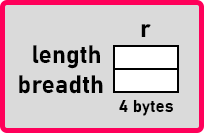This ‘r’ is created inside the stack. Now if we write,

Rectangle *p;

This is also created inside the stack, then if we say,

p = &r;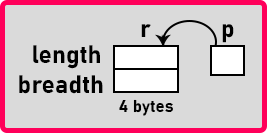So here, the ‘p’ pointer will be pointing to the ‘r’ rectangle object. Now we want to access the length to store 10. So, using the ‘r’ object, I can say

r.length =10;

You already know how to access the members of an object using the dot operator. But now we want to access this using pointer. We can say,

p->length = 10;

In our discussion, we will say ‘->’ as a caps or arrow operator. So, you have to use these two symbols i.e. hyphen and greater than sign for forming this arrow symbol. The dot operator is used for accessing the members of an object using a variable name and the arrow operator is used for accessing the members of an object using a pointer on an object.

We can access anything like ‘p->breadth = 4;’ and 4 are stored in breadth then we can say,

cout << p->Area();

Here area will be displayed. So, this arrow ‘->’ is a dereferencing operator. Instead of using a star, we can use this arrow. We may be calling it an arrow or caps. This can be used for accessing the members of an object. You can access the data members as well as functions.

##### How to create an object in heap using a pointer in C++?

Now let’s see how to create an object in heap using a pointer. If we write,

Rectangle *p;

We know that the pointer will be created inside the stack. What is the size of this pointer? How many bytes it will take? As per our assumptions, this pointer will take 2 bytes only. Actually, it depends on the compiler, it may take four bytes also. So, we are saying that it takes 2 bytes, so all the pointer takes 2 bytes i.e. integer pointer or character pointer, or any pointer.

Now, the ‘p’ pointer is declared but the object is not created then write,

p = new Rectangle;

Now objects will be created with length and breadth and the ‘p’ pointer will be pointing at them.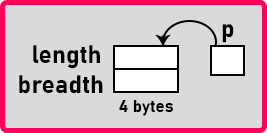##### Where this object is created?

This object will be created in heap. See first we declared a pointer then we assigned that to the new Rectangle then an object will be created in the heap memory. We can write the whole thing in a single line also as

Rectangle *q = new Rectangle();

Even we can write empty brackets here. So, q is a pointer assigned to a new Rectangle. We have now another object created inside the heap memory with length and breadth.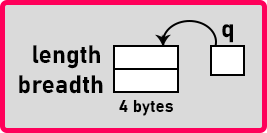So, there is no name to the object but pointer ‘q’ is pointing onto that one. This is the method of creating an object in heap memory. These are the two methods to create an object in the stack and to create an object in the heap memory. Now one thing that in Java you cannot create an object in the stack, always objects are created in the heap memory by only using the ‘new’ keyword but C++ gives you an option whether you can create an object in the stack or whether you want it in heap.

You decide, so what kind of performance you want, what kind of requirements you have based on that you can use any one of these methods. So, C++ gives more options to the programmer. Now let us write the complete program.

##### Program to show How to Create Objects in Heap using C++
```#include <iostream>
using namespace std;

class Rectangle
{
public:
int length;

int Area ()
{
}
int Perimeter ()
{
return 2 * (length + breadth);
}
};

int main()
{
Rectangle *p, temp;		// Inside Stack
p = &temp;
p->length = 10;

Rectangle *q = new Rectangle ();	// Inside Heap
q->length = 3;

cout << "p:" << endl;
cout << "length: " << p->length << " breadth: " << p->breadth << endl;
cout << "q:" << endl;
cout << "length: " << q->length << " breadth: " << q->breadth << endl;

cout << "Area of p: " << p->Area () << endl;
cout << "Perimeter of p: " << p->Perimeter () << endl << endl;
cout << "Area of q: " << q->Area () << endl;
cout << "Perimeter of q: " << q->Perimeter () << endl;
}
```
###### Output: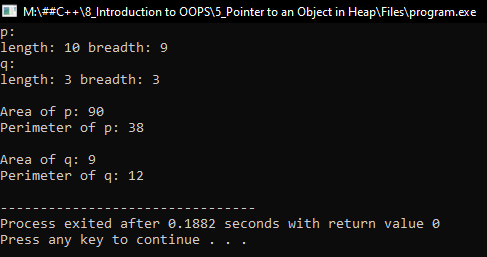1.